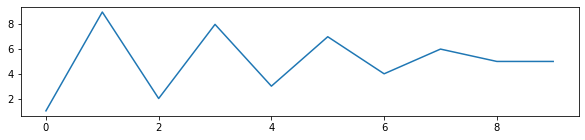👋 Hello! I'm Alphonsio the robot. Ask me a question, I'll try to answer.

# In Python, how do you change the size of an already created figures drawn with Matplotlib?

The simplest way to change the size of an existing Matplotlib figure is to use the method ﻿set_size_inches():

import matplotlib.pyplot as plt
plt.plot ([1,9,2,8,3,7,4,6,5,5])

# Set figure size in inches
fig = plt.gcf()
fig.set_size_inches(20, 4)

The above code should display something like:More